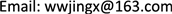1南京电子技术研究所，江苏 南京

2南京航空航天大学，江苏 南京1. 引言

2. 系统型和设计目标2.1. 系统模型

• Gen(λ)→K：用户根据安全参数λ生成密钥K，用于后续的加密和解密过程。

• Enc(K, X)→X'：用户利用密钥K将私有输入X加密成X'。

• Compute(X')→Y'：云服务器计算矩阵X'的逆矩阵Y'。

• Verify(Y')→0/1：用户验证云服务器返回值Y'的正确与否，1表示正确，0表示不正确。

• Dec(K, Y')→Y：用户在验证Y'正确的情况下，利用K解密Y'得到矩阵X的逆矩阵Y。

2.2. 设计目标

1) 输入隐私性：安全外包方案需要保护用户的输入数据的隐私性，即矩阵X是用户的隐私数据，在方案执行前后都不能泄漏给云服务器或任何第三方。

2) 输出隐私性：用户的输出结果Y也是隐私的，其隐私性需要有效保护，云服务器不能获得Y的有用信息。

3) 效率：用户端总的计算开销应该远小于直接矩阵求逆所需要的开销，即安全外包可以降低用户的的计算开销。

4) 正确性：用户能够以较高的概率验证云服务器返回的结果正确与否。如果云服务器返回的Y'是错误的，用户能够以很高的概率检测出不正确的输出；如果云服务器返回的的Y'是正确的，Y'能够通过用户的验证。

3. 方案设计3.1. 主要思路

Y = D R Y ′ D L .

det [ E F G H ] = det ( H ) ∗ det ( E − F H − 1 G ) .

det ( D L ) = ( 1 + P 1 L 1 + ∑ i = 2 n P i Q i L i ) ∗ ∏ j = 1 n L j ,

det ( D R ) = ( 1 + U 1 R 1 + ∑ i = 2 n U i V i R i ) ∗ ∏ j = 1 n R j ,

D L = [ P 1 + L 1 Q 2 P 1 ⋯ Q n P 1 P 2 Q 2 P 2 + L 2 ⋯ Q n P 2 ⋮ ⋮ ⋱ ⋮ P n Q 2 P n ⋯ Q n P n + L n ] .

det ( D L ) = det [ P 1 + L 1 − Q 2 L 1 ⋯ − Q n L 1 P 2 L 2 ⋯ 0 ⋮ ⋮ ⋱ ⋮ P n 0 ⋯ L n ] .

det ( D L ) = det [ E F G H ] = det ( H ) ∗ det ( E − F H − 1 G ) .

det ( D L ) = ( P 1 + L 1 + ∑ i = 2 n P i Q i D 1 L i ) ∗ ∏ j = 2 n L j = ( 1 + P 1 L 1 + ∑ i = 2 n P i Q i L i ) ∗ ∏ j = 1 n L j = det ( D L ) = ( 1 + Q L − 1 P ) ∗ det ( L ) .

1) 对于i = 1到n，随机选择非零的Li和Ri

2) 设定 Q 1 = V 1 = 1 ，并对于i = 2到n，随机选择非零的{Pi, Qi}和{Ui, Vi}；然后随机选择P1和U1，使得 且 U 1 ≠ − R 1 ∗ ( 1 + ∑ i = 2 n U i V i R i ) 。

3.2. 方案步骤

• Gen：用户1) 随机生成n × n对角矩阵L和R，其对角线元素依次分别是 ( L 1 , L 2 , ⋯ , L n ) 和 ( R 1 , R 2 , ⋯ , R n ) ，并且满足任意的Li和Ri都不等于0。2) 对于I = 2到n，分别随机选择非零的{Pi, Qi}和{Ui, Vi}；然后随机选择P1和U1，使得 P 1 ≠ − L 1 ∗ ( 1 + ∑ i = 2 n P i Q i L i ) 且 U 1 ≠ − R 1 ∗ ( 1 + ∑ i = 2 n U i V i R i ) 。3) 设定矩阵 P = ( P 1 , P 2 , ⋯ , P n ) T ， U = ( U 1 , U 2 , ⋯ , U n ) T ， Q = ( 1 , Q 2 , ⋯ , Q n ) ， V = ( 1 , V 2 , ⋯ , V n ) 。4) 将密钥K设置为 K = { L , P , Q , R , U , V } 。

• Enc：用户利用密钥K将私有输入X加密成X'，使得 X ′ = ( L + P Q ) X ( R + U V ) 。

• Compute：用户将加密后的矩阵X'发送给云服务器。云服务器计算矩阵X'的逆矩阵Y'，并将矩阵Y'返回给用户。

• Verify：用户随机生成n × 1的矩阵 S = ( S 1 , S 2 , ⋯ , S n ) T ，然后计算 S ′ = X ′ ( Y ′ S ) 。用户比较S'和S，如果 S'=S，则返回值Y'通过验证；否则Y'没通过验证。

• Dec：在验证Y'正确的情况下，用户利用密钥K解密云服务器返回的矩阵Y'，得到矩阵 Y = ( R + U V ) Y ′ ( L + P Q ) 。

4. 方案分析

4.1. 理论分析

det ( L + P Q ) = ( 1 + P 1 L 1 + ∑ i = 2 n P i Q i L i ) ∗ ∏ j = 1 n L j ,

det ( R + U V ) = ( 1 + U 1 R 1 + ∑ i = 2 n U i V i R i ) ∗ ∏ j = 1 n R j ,

4.2. 实验评估

Running time of simulation experiment

1001.0020.0190.99952.737
500197.8090.663200.121298.354
10001103.0511.8391089.476599.810
20009000.2137.0029060.0921285.377
300029108.17213.59830001.0442140.622
5000136110.83035.275138029.4253858.564

5. 结束语